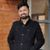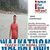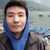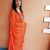# How can a positive NPV end up with negative NPV with the same set of cash flow and time horizon

Provide an example of a project with positive NPV and a 5-year time horizon. Demonstrate what can be changed in the example to end up with negative NPV if we use the same set of cash flows and same time horizon?

This post was part of TyroCity discussion forumAngel Paudel

Net present value (NPV) in financial term refers to the measurement of profit calculated by subtracting the present value of cash outflow which includes of the initial cost and further funneling of funds into the project from the present values of cash inflows over the set period of time (Ljubić, Mrša & Stanković, 2013).

As per the given criteria, to look into a project with positive Net Present Value (NPV) these details are taken into account/consideration:

Total initial investment: NRs. 5,00,000
Discount Rate: 5%
Timeframe: 5 years

Another assumption made for the calculation is that no further investment has been made within the five years taken into consideration. The cash flow is considered to be even and of NRs. 1,25,000 every year for five years consistently. The discount rate is considered to be fixed and consistent for every year taken into account. The value within brackets is to represent that they denote negative value.

Here’s the calculation for the NPV on the above set parameters:

Year Cash flow (CF) Present Value (PV) = [1/(1+i)^n] Discounted cash flow (CF*PV)
0 (500000) 1 (500000)
1 125000 0.95 118750
2 125000 0.91 113750
3 125000 0.86 107500
4 125000 0.82 102500
5 125000 0.78 97500
NPV 40000

In this case, we obtained a positive NPV which is NRs. 40,000. However, to end up with a negative NPV while using the same cash flow and time horizon, the discount rate should be varied. All the other parameters are considered to be the same except for the discount rate which is now considered to be 10% instead of 5%. Here’s the calculation with the updated discount rate:

Year Cash flow (CF) Present Value (PV) = [1/(1+i)^n] Discounted cash flow (CF*PV)
0 (500000) 1 (500000)
1 125000 0.91 113750
2 125000 0.83 103750
3 125000 0.75 93750
4 125000 0.68 85000
5 125000 0.62 77500
NPV (26250)

As we can see from the demonstration, with the change in the discount rate, we end up with a negative NPV of NRs. 26,250.

With these demonstrations, we can conclude that we can end up with negative NPV while keeping the same cash flow and time horizon with just change made to the discount rate.

References

Ljubić, D., Mrša, J., & Stanković, S. (2013). Interaction of Net Present Value, Cash Flow and Financial Statements. SSRN Electronic Journal , 1-2.Net present value is the present value of the cash flows at the required rate of return of your project compared to your initial investment,” says Frank Knight (1921). In practical terms, it’s a method of calculating your return on investment, or ROI, for a project or expenditure. By looking at all of the money you expect to make from the investment and translating those returns into today’s Prices, you can decide whether the project is worthwhile

Net present value is based on the concept of equivalent worth of all cash flows to the base point or beginning point in time called the present (Gallo A. , 2016). Net present value (NPV) of a given series of cash flow is the equivalent value/worth at the end of year zero or beginning of year 1. Hence, according to this method all cash inflows and outflows are discounted to the base point at an interest rate for the economic study period in the following manner.

PW(i) = F0(1 + i)0 + F1(1 + i)-1 + F2(1 + i)-2 +…+ FN(1 + i)-N

PW(i) = ∑NK=0 FK (1 + i)-k

Where, i= Effective interest rate or MARR per compounding periods

K= Index for each compounding periods
Fk= Future Cash flow at the end of period K
N = Number of compounding periods in economic study.

Decision Rule

While using the Net Present Value method for economic evaluation of any project, we should consider the following decision rule.

If PW (i) > 0, accept the Project

If PW (i) = 0, remain indifference

If PW (i) < 0, reject the Project

Example (1):

Initial Investment (I) = Rs. 1000000
Annual Revenue ® = Rs. 20000
Annual Expenses (E) = Rs. 5000
Salvage Value (S) = Rs. 25000
Useful Lifecycle = Rs. 5 years
MARR = 10% per year.

Using Net Present value formulation

PW (10%) = -100000 + (20000 - 5000) (P/A, 10%, 10) + 25000 (P/F, 10%, 10)

PW (10%) = -100000 + 15000[(1 + i)N - 1/i(1 + i)N] + 25000 [(1 + i)-N ]

PW (10%) = -100000 + 15000[(1 + 0.1)5 - 1/i(1 + 0.1)5] + 25000 [(1 + 0.1)-5 ]

PW (10%) = Rs. 1807

Since, PW (10%) > 0, the project is acceptable for investment.

Similarly, for other example (2) is

Land cost = Rs. 10, 00000
Building cost = Rs. 1200000
Equipment = Rs. 400000
Sales revenue = Rs. 1500000 per years for 5 years

Salvage values after 5 years will be as follows.
Land = Rs. 2000000
Building = Rs 300000
Equipment = Rs 100000
Annual expenses = Rs. 1000000
MARR = 20%

The Total investment = Rs. (1000000 + 1200000 + 400000) = 2600000
Net annual revenue = Rs. (1500000 - 1000000) = Rs. 500000
Salvage Value = Rs. (2000000 + 300000 + 100000) = Rs. 2400000

Using Net Present value

PW(20%) = - 2600000+ 500000 (P/A, 20%, 5) + 2400000 (P/F, 20%.5)
PW(20%) = - 2600000+ 500000 (4.19247) + 2400000 (0.1615)
PW(20%) = - 2600000 + 2096235 + 387600
PW(20%) = -116165

Since, PW(20%) is negative (i.e. < 0), The proposed plan is rejected to investment.

We can conclude that when we increase the discounted rate of project then the value of Net Present Value is negative. We can clearly visualize from Example (1) and Example (2). More the Discounted rate the PW may be negative and then the proposed plan is rejected. But less the discounted rate the PW may be positive and then the proposed plan is accepted. Higher the rate of IRR the maximum chance to come negative value of present worth in our purposed plan and similarly lower the rate of IRR the maximum chance to come positive value of present value in our purpose plant. So when the NPV comes in positive value then only then purposed plan is accepted. The discount rate will be company-specific as it’s related to how the company gets its funds. It’s the rate of return that the investors expect or the cost of borrowing money. If shareholders expect a 12% return that is the discount rate the company will use to calculate NPV. If the firm pays 4% interest on its debt, then it may use that figure as the discount rate. At this condition the ROI rate is higher than discounted rate so Net Present value is Positive.

There are generally three options available: internal rate of return, payback method, and net present value.Frank Knight (1921) says that net present value, often referred to as NPV, is the tool of choice for most financial analysts. There are two reasons for that. One, NPV considers the time value of money, translating future cash flows into today’s Prices.

References
Frank, K. (1993). Risk, Uncertainty, and Profit. Boston: Houghton Mifflin.

Gallo, A. (2016). A Refresher on Net Present Value. Harvard Business Review.Net Present Value (NPV) has practical implication and so widely used in business decision making as it includes the time value of money. This theoretical method has been found to be used 80% in business decision for new expansion and 60% for mutually exclusive projects. Its use has increased over the years for capital budgeting and financial decision making. (Sun & Queyranne, 2002) Here we will look into how positive and negative NPV are generated and what is the driving factor for this.

Let us take the following for a project.

Initial investment or Present value of cash outflow = Rs. 250,000.00

Discounted Rate of return = 10 %

Period = 5 years

Expected annual cash inflow = Rs. 70,000

In this example we are taking an example of a consistent cash flow only. Let us calculate the discounted cash inflow and net present value.

Figure 1:

A B C=A*B D=D-C
Year Cash Flow (CF) in Rs. Present Value (PV ) = 1/(1+i)^n PV of Cash inflow (in Rs) = CF * PV NPV = PV of Cash Inflow – Cash outflow (In Rs)
0 (250,000) 1 (250,000) (250,000)
1 70,000 0.9091 63,637 (186,363)
2 70,000 0.8264 57,848 (128,515)
3 70,000 0.7513 52,591 (75,924)
4 70,000 0.6830 47,810 (28,114)
5 70,000 0.6209 43,463 15,349

Here we can see that the project can be selected as it gives a positive value in the last year.

Now let us consider the same project with the same initial outflow, period, and expected cash inflow but increase the discounted rate of return to 20%.

Figure 2:

A B C=A*B D=D-C
Year Cash Flow (CF) in Rs. Present Value (PV ) = 1/(1+i)^n PV of Cash inflow (in Rs) = CF * PV NPV = PV of Cash Inflow – Cash outflow (In Rs)
0 (250,000) 1 (250,000) (250,000)
1 70,000 0.8333 58,331 (191,669)
2 70,000 0.6944 48,608 (143061)
3 70,000 0.5787 40,509 (102552)
4 70,000 0.4823 33,761 (68,791)
5 70,000 0.4019 28,133 (40,658)

In Figure 2 we can see that the NPV is still negative in the fifth year with a discounted rate of return of 20% but a positive NPV is reflected in figure 1 with a discounted rate of 10%. What this shows is that the investment in figure 2 should be rejected while the investment in figure 1 should be selected as it has positive cash flow at the end of the expected year.

From this we deduce that a higher discounted rate of return is not favourable for investment.

Reference

Sun, D., & Queyranne, M. (2002). Production and inventory model using net present value. Operations Research; Linthicum , 528-537. Retrieved from proxy.lirn.net/MuseProxyID=mp02/Mu...Net Present Value (NPV) is the difference between total present value of cash inflows and outflows. It iswidely used method for evaluating the desirability of the project as it considers the time value of money as well as timing of the cash flows (Moyer, McGuigan, Rao, & Kretlow, 2012).

Calculation of NPV

For the calculation of NPV, let us assume that the initial investment is Rs. 2,000,000 and the discount rate is 20%. In addition, the cash flows are uneven and no additional investments are made in following years.

Year Cash flow (CF) PV Factor @ 20% Discounted CF
1 800,000 0.8333 666,640
2 700,000 0.6944 486,080
3 650,000 0.5787 376,155
4 600,000 0.4823 289,380
5 800,000 0.4019 321,520
Total 2,139,775
Less: Initial Investment 2,000,000
NPV 139,775

If we increase the discount rate to 25% keeping the cash flows and time horizon constant, the NPV will be:

Year Cash flow (CF) PV Factor @ 25% Discounted CF
1 800,000 0.8000 640,000
2 700,000 0.6400 448,000
3 650,000 0.5120 332,800
4 600,000 0.4096 245,760
5 800,000 0.3277 262,160
Total 1,928,720
Less: Initial Investment 2,000,000
NPV -71,280

From the two different cases, we can see that the increase in discount rate leads to decrease in NPV of a project keeping other variables constant. In case of independent projects, the projects with positive NPV are accepted and project with highest NPV among the given alternatives is selected if the projects are mutually exclusive. Thus the projects with lower discount rate are more likely to be accepted as it results in higher NPV.

References
Moyer, R. C., McGuigan, J. R., Rao, R., & Kretlow, W. J. (2012). Contemporary Financial Management (12th ed.). Oklahoma: Cengage Learning.